# Extremely simple yet powerful header-only C++ plotting library built on the popular matplotlib

### Related tags

Math matplotlib-cpp

# matplotlib-cpp

Welcome to matplotlib-cpp, possibly the simplest C++ plotting library. It is built to resemble the plotting API used by Matlab and matplotlib.

## Usage

Complete minimal example:

```#include "matplotlibcpp.h"
namespace plt = matplotlibcpp;
int main() {
plt::plot({1,3,2,4});
plt::show();
}```
``````g++ minimal.cpp -std=c++11 -I/usr/include/python2.7 -lpython2.7
``````

Result:A more comprehensive example:

```#include "matplotlibcpp.h"
#include <cmath>

namespace plt = matplotlibcpp;

int main()
{
// Prepare data.
int n = 5000;
std::vector<double> x(n), y(n), z(n), w(n,2);
for(int i=0; i<n; ++i) {
x.at(i) = i*i;
y.at(i) = sin(2*M_PI*i/360.0);
z.at(i) = log(i);
}

// Set the size of output image to 1200x780 pixels
plt::figure_size(1200, 780);
// Plot line from given x and y data. Color is selected automatically.
plt::plot(x, y);
// Plot a red dashed line from given x and y data.
plt::plot(x, w,"r--");
// Plot a line whose name will show up as "log(x)" in the legend.
plt::named_plot("log(x)", x, z);
// Set x-axis to interval [0,1000000]
plt::xlim(0, 1000*1000);
plt::title("Sample figure");
// Enable legend.
plt::legend();
// Save the image (file format is determined by the extension)
plt::save("./basic.png");
}```
``````g++ basic.cpp -I/usr/include/python2.7 -lpython2.7
``````

Result:Alternatively, matplotlib-cpp also supports some C++11-powered syntactic sugar:

```#include <cmath>
#include "matplotlibcpp.h"

using namespace std;
namespace plt = matplotlibcpp;

int main()
{
// Prepare data.
int n = 5000; // number of data points
vector<double> x(n),y(n);
for(int i=0; i<n; ++i) {
double t = 2*M_PI*i/n;
x.at(i) = 16*sin(t)*sin(t)*sin(t);
y.at(i) = 13*cos(t) - 5*cos(2*t) - 2*cos(3*t) - cos(4*t);
}

// plot() takes an arbitrary number of (x,y,format)-triples.
// x must be iterable (that is, anything providing begin(x) and end(x)),
// y must either be callable (providing operator() const) or iterable.
plt::plot(x, y, "r-", x, [](double d) { return 12.5+abs(sin(d)); }, "k-");

// show plots
plt::show();
}```
``````g++ modern.cpp -std=c++11 -I/usr/include/python2.7 -lpython
``````

Result:Or some funny-looking xkcd-styled example:

```#include "matplotlibcpp.h"
#include <vector>
#include <cmath>

namespace plt = matplotlibcpp;

int main() {
std::vector<double> t(1000);
std::vector<double> x(t.size());

for(size_t i = 0; i < t.size(); i++) {
t[i] = i / 100.0;
x[i] = sin(2.0 * M_PI * 1.0 * t[i]);
}

plt::xkcd();
plt::plot(t, x);
plt::title("AN ORDINARY SIN WAVE");
plt::save("xkcd.png");
}
```
``````g++ xkcd.cpp -std=c++11 -I/usr/include/python2.7 -lpython2.7
``````

Result:When working with vector fields, you might be interested in quiver plots:

```#include "../matplotlibcpp.h"

namespace plt = matplotlibcpp;

int main()
{
// u and v are respectively the x and y components of the arrows we're plotting
std::vector<int> x, y, u, v;
for (int i = -5; i <= 5; i++) {
for (int j = -5; j <= 5; j++) {
x.push_back(i);
u.push_back(-i);
y.push_back(j);
v.push_back(-j);
}
}

plt::quiver(x, y, u, v);
plt::show();
}```
``````g++ quiver.cpp -std=c++11 -I/usr/include/python2.7 -lpython2.7
``````

Result:When working with 3d functions, you might be interested in 3d plots:

```#include "../matplotlibcpp.h"

namespace plt = matplotlibcpp;

int main()
{
std::vector<std::vector<double>> x, y, z;
for (double i = -5; i <= 5;  i += 0.25) {
std::vector<double> x_row, y_row, z_row;
for (double j = -5; j <= 5; j += 0.25) {
x_row.push_back(i);
y_row.push_back(j);
z_row.push_back(::std::sin(::std::hypot(i, j)));
}
x.push_back(x_row);
y.push_back(y_row);
z.push_back(z_row);
}

plt::plot_surface(x, y, z);
plt::show();
}```

Result:## Installation

matplotlib-cpp works by wrapping the popular python plotting library matplotlib. (matplotlib.org) This means you have to have a working python installation, including development headers. On Ubuntu:

``````sudo apt-get install python-matplotlib python-numpy python2.7-dev
``````

If, for some reason, you're unable to get a working installation of numpy on your system, you can define the macro `WITHOUT_NUMPY` before including the header file to erase this dependency.

The C++-part of the library consists of the single header file `matplotlibcpp.h` which can be placed anywhere.

Since a python interpreter is opened internally, it is necessary to link against `libpython` in order to user matplotlib-cpp. Most versions should work, although python likes to randomly break compatibility from time to time so some caution is advised when using the bleeding edge.

# CMake

The C++ code is compatible to both python2 and python3. However, the `CMakeLists.txt` file is currently set up to use python3 by default, so if python2 is required this has to be changed manually. (a PR that adds a cmake option for this would be highly welcomed)

NOTE: By design (of python), only a single python interpreter can be created per process. When using this library, no other library that is spawning a python interpreter internally can be used.

To compile the code without using cmake, the compiler invocation should look like this:

``````g++ example.cpp -I/usr/include/python2.7 -lpython2.7
``````

This can also be used for linking against a custom build of python

``````g++ example.cpp -I/usr/local/include/fancy-python4 -L/usr/local/lib -lfancy-python4
``````

# Vcpkg

``````git clone https://github.com/Microsoft/vcpkg.git
cd vcpkg
./bootstrap-vcpkg.sh
./vcpkg integrate install
vcpkg install matplotlib-cpp
``````

The matplotlib-cpp port in vcpkg is kept up to date by Microsoft team members and community contributors. If the version is out of date, please create an issue or pull request on the vcpkg repository.

# C++11

Currently, c++11 is required to build matplotlib-cpp. The last working commit that did not have this requirement was `717e98e752260245407c5329846f5d62605eff08`.

Note that support for c++98 was dropped more or less accidentally, so if you have to work with an ancient compiler and still want to enjoy the latest additional features, I'd probably merge a PR that restores support.

## Why?

I initially started this library during my diploma thesis. The usual approach of writing data from the c++ algorithm to a file and afterwards parsing and plotting it in python using matplotlib proved insufficient: Keeping the algorithm and plotting code in sync requires a lot of effort when the C++ code frequently and substantially changes. Additionally, the python yaml parser was not able to cope with files that exceed a few hundred megabytes in size.

Therefore, I was looking for a C++ plotting library that was extremely easy to use and to add into an existing codebase, preferably header-only. When I found none, I decided to write one myself, which is basically a C++ wrapper around matplotlib. As you can see from the above examples, plotting data and saving it to an image file can be done as few as two lines of code.

The general approach of providing a simple C++ API for utilizing python code was later generalized and extracted into a separate, more powerful library in another project of mine, wrappy.

## Todo/Issues/Wishlist

• This library is not thread safe. Protect all concurrent access with a mutex. Sadly, this is not easy to fix since it is not caused by the library itself but by the python interpreter, which is itself not thread-safe.

• It would be nice to have a more object-oriented design with a Plot class which would allow multiple independent plots per program.

• Right now, only a small subset of matplotlibs functionality is exposed. Stuff like xlabel()/ylabel() etc. should be easy to add.

• If you use Anaconda on Windows, you might need to set PYTHONHOME to Anaconda home directory and QT_QPA_PLATFORM_PLUGIN_PATH to %PYTHONHOME%Library/plugins/platforms. The latter is for especially when you get the error which says 'This application failed to start because it could not find or load the Qt platform plugin "windows" in "".'

• MacOS: `Unable to import matplotlib.pyplot`. Cause: In mac os image rendering back end of matplotlib (what-is-a-backend to render using the API of Cocoa by default). There is Qt4Agg and GTKAgg and as a back-end is not the default. Set the back end of macosx that is differ compare with other windows or linux os. Solution is described here, additional information can be found there too(see links in answers).

• #### segmentation fault when linking against python3

The library builds fine when linking against python3 but has a segmentation fault when program terminates. Maybe the solution is to have a static assert somewhere when the python version used isn't 2.7

opened by pfeatherstone 6
• #### Adds axvline, boxplot to function scope (et al.)

Title says it all. Other than that a bit of extra whitespace got remove, I'm afraid, sorry about that.

I tried to keep with your codestyle best I could, if there's anything you would like changed let me know (or make the change yourself, of course).

Also, I'm not sure I stayed in line with how you like functions to be called, i.e. this could've been done as a template on `Numeric` but I didn't really see any benefit in it, I may be wrong here.

ALSO, and lastly, I would understand if you would prefer I add also `avyline` before merging this. Let me know.

opened by cdbrkfxrpt 6
• #### Moving from std::vector to templated vectors

I've run into this while preparing a lecture using not `std::vector<T>` but another vector type from the Eigen library. The plot functions used in matplotlib-cpp are all templated as

``````template <typename Numeric>
bool a_plot_function(const std::vector<Numeric>& x, ...)
``````

and it is no trouble to rewrite it as

``````template <typename Vector>
bool a_plot_function(const Vector& x, ...)
``````

This would enable the usage of the widely-used Eigen library (at least in numerical analysis) and also other generic vector classes, implementing the methods of STL vectors.

@lava I have already implemented this, however my fork has a few more changes than this one only, therefore I wanted to discuss this before opening a PR. What do you think of this extension?

opened by Cryoris 6
• #### Using the plt::contour() function leads to a compilation error: ‘get_array’ was not declared in this scope

I would like to use a contour map for plotting a compressor map but unfortunately I'm not able to use the plt::contour() function since the following error occurs:

``````In file included from ../main.cpp:2:0:
../include/matplotlibcpp.h: In instantiation of ‘bool matplotlibcpp::contour(const std::vector<Numeric>&, const std::vector<NumericY>&, const std::vector<XType>&, const std::map<std::__cxx11::basic_string<char>, std::__cxx11::basic_string<char> >&) [with NumericX = std::vector<double>; NumericY = std::vector<double>; NumericZ = std::vector<double>]’:
../main.cpp:211:37:   required from here
../include/matplotlibcpp.h:1142:33: error: ‘get_array’ was not declared in this scope
PyObject* xarray = get_array(x);
~~~~~~~~~^~~
../include/matplotlibcpp.h:1142:33: note: suggested alternative:
In file included from ../main.cpp:2:0:
../include/matplotlibcpp.h:403:19: note:   ‘matplotlibcpp::detail::get_array’
inline PyObject * get_array(const std::vector<std::string>& strings)
^~~~~~~~~
In file included from ../main.cpp:2:0:
../include/matplotlibcpp.h:1143:33: error: ‘get_array’ was not declared in this scope, and no declarations were found by argument-dependent lookup at the point of instantiation [-fpermissive]
PyObject* yarray = get_array(y);
~~~~~~~~~^~~
../include/matplotlibcpp.h:1142:33: note: ‘get_array’ declared here, later in the translation unit
PyObject* xarray = get_array(x);
~~~~~~~~~^~~
../include/matplotlibcpp.h:1144:33: error: ‘get_array’ was not declared in this scope, and no declarations were found by argument-dependent lookup at the point of instantiation [-fpermissive]
PyObject* zarray = get_array(z);
~~~~~~~~~^~~
../include/matplotlibcpp.h:1142:33: note: ‘get_array’ declared here, later in the translation unit
PyObject* xarray = get_array(x);
~~~~~~~~~^~~
``````

I use the python 3.6 flags because since the newest commit I cant use python 2.7 anymore because I get the following compiler error: `cannot convert ‘wchar_t**’ to ‘char**’` like in this issue #225

opened by shyney7 5
• #### exception "access violation" with minimal example

I'm getting an exception with access violation with the minimal example, change slightly to that below, see screenshot for place of exception (plot->first detail::get_array(X) -> PyList_New(v.size());

Any idea what is wrong?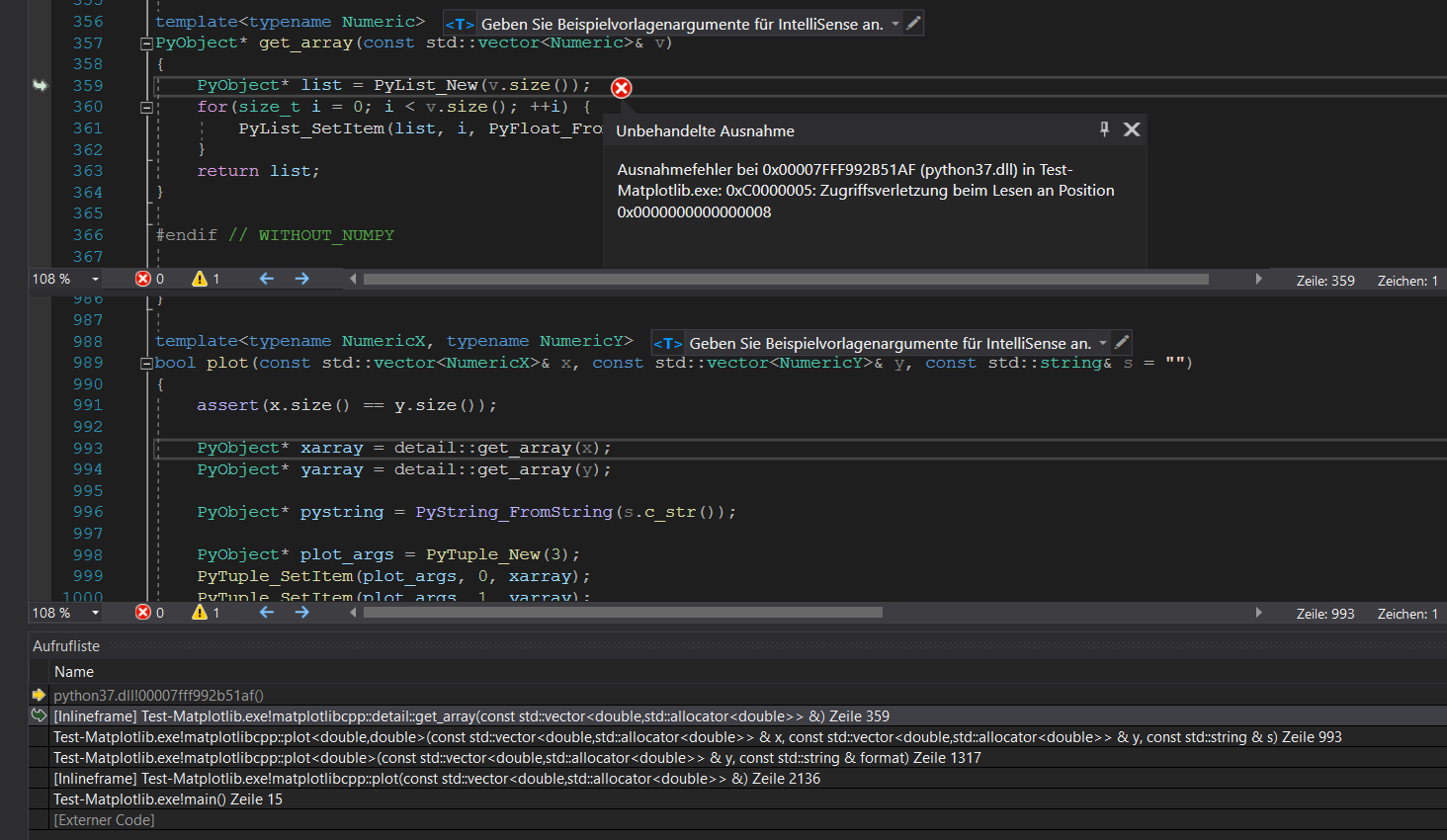#include // according to: https://github.com/boostorg/system/issues/32 #define HAVE_SNPRINTF #include <Python.h>

#define WITHOUT_NUMPY #include "C:\Users\tsch\OneDrive\Dokumente\fiberdesk wo code\fiberdesk code dependencies\matplotlib-cpp-master\matplotlibcpp.h"

int main() { std::cout << "Hello world"; namespace plt = matplotlibcpp;

``````plt::plot({ 1,3,2,4 });

return 0;
``````

}

opened by TomFD 5

Hello. I have seen this issue happening for mac OS users but I'm currently on windows10 and I don't know how to fix this.

I'm using Visual Studio 2017, and Python 2.7. However, I also have Python 3.7 and Anaconda in my PC, so I hope this is not messing up the linker to the proper libraries.

In matplotlibcpp.h , line 137. I'm getting an exception:

Excepción producida en 0x00007FFFCF28E78A (ntdll.dll) en ConsoleApplication2.exe: 0xC0000008: An invalid handle was specified.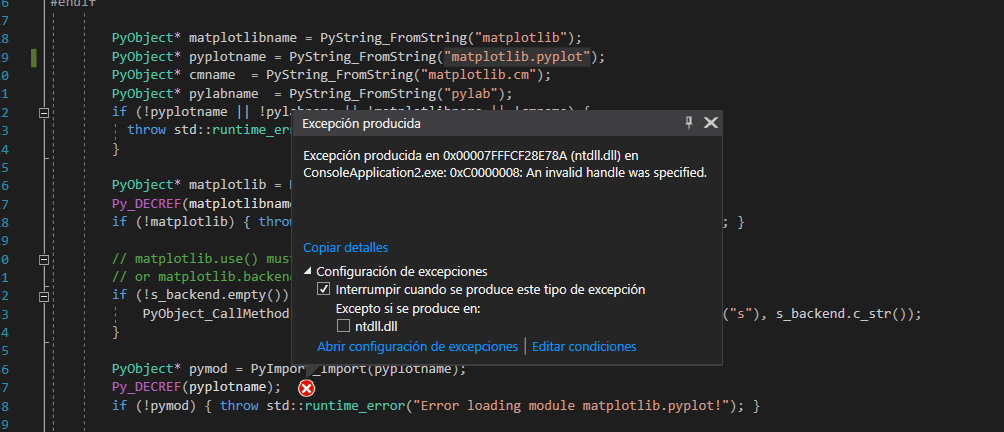When I type import matplotlib.pyplot in the terminal (using python2.7), it works perfectly.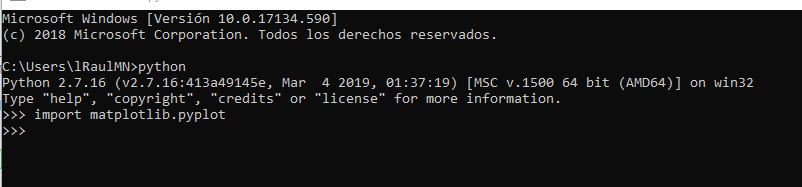If I manipulate the name in line 119, so instead of "matplotlib.pyplot" I write "matplotlib/pyplot", I reach the line 138 and it pop's up the error message as expected.

So I assume it's finding the module but once he opens it, there is an error. Any ideas? I have seen a lot of post about this for Mac, but none for Windows.

opened by lRaulMN7 5
• #### Add basic support for quiver function

This commit adds support for the quiver function including the option to add keywords. At present, there is only the option to pass vectors of x, y, u and v vectors of equal length, but I might add support for some of the other overloads at a later stage.

Anyway, this version is sufficient for my use case and hopefully it'll be handy for someone else too!

opened by alexdewar 5
• #### Adding support for axvspan issue

I'm trying to add support for axvspan. I've done the following:

• added: `s_python_function_axvspan`
• added: `s_python_function_axvspan = safe_import(pymod, "axvspan");`
``````inline void axvspan(double xmin, double xmax, double ymin = 0., double ymax = 1., const std::map<std::string, std::string>& keywords = std::map<std::string, std::string>())
{
// construct positional args
PyObject* args = PyTuple_New(3);
PyTuple_SetItem(args, 0, PyFloat_FromDouble(xmin));
PyTuple_SetItem(args, 1, PyFloat_FromDouble(xmin));
PyTuple_SetItem(args, 2, PyFloat_FromDouble(ymin));
PyTuple_SetItem(args, 3, PyFloat_FromDouble(ymax));

// construct keyword args
PyObject* kwargs = PyDict_New();
for(std::map<std::string, std::string>::const_iterator it = keywords.begin(); it != keywords.end(); ++it)
{
PyDict_SetItemString(kwargs, it->first.c_str(), PyString_FromString(it->second.c_str()));
}

PyObject* res = PyObject_Call(detail::_interpreter::get().s_python_function_axvspan, args, kwargs);

Py_DECREF(args);
Py_DECREF(kwargs);

if(res) Py_DECREF(res);
}
``````

This function has no effect on the graph and the res pointer return is always NULL. Any idea why this may be?

opened by pfeatherstone 4
• #### Add support for imshow()

This PR adds support for the imshow() function to matplotlibcpp. imshow() displays an image in the current figure and allows for setting extra parameters such as the colormap used.

I've also added a version of the function which takes an OpenCV matrix as an input (in a separate commit), which provides a nicer interface. This requires OpenCV, but I've made this functionality optional: you have to explicitly define WITH_OPENCV if you want it.

opened by alexdewar 4
• #### Added CMake support for library

The purpose of this PR is to integrate CMake support directly to project and preserve the current Makefile-correctness at the same time. The reason for adding CMakeLists.txt is to be able to integrate this library in other projects using add_subdirectory() feature in CMake. Also, CMakeLists.txt for this library is desired as it provides include & link settings for library which would one have to add manually in his project.

This PR has been tested on both Linux (Fedora, gcc/clang) and Windows (VS).

opened by Romop5 4
• #### Implement STL-like interface for plotting generic data structures

This PR is motivated by the desire to plot data in generic data structures without the boilerplate of copying data into `std::vectors` before each plot. (I just had to plot a 101385-element Eigen::VectorXd and the copy was NOT fun).

The solution adopted is to follow the example of the STL and define the graph data by a pair of templated input iterators. For ease of use, the interface is designed to mimic the STL in the following manner.

``````std::transform(a.begin(), a.end(), b.begin(), binaryFunctor);
plt::stl::plot(a.begin(), a.end(), b.begin(), "ro");
``````

only 2D lineplots and scatter plots are addressed for the time being.

In due diligence, the examples `stl_containers.cpp` and `eigen_objects.cpp` and a section reviewing this interface in the README are added.

I think the main sticking points regarding this PR are

1. If the STL-like interface looks out of place among functions mimicking matplotlib's interface
2. The mechanism handling Contiguous and Non-Contiguous containers is clunky.

I suppose C++20 `<ranges>` is the silver bullet to resolve point 1, and C++20 `contiguous_iterator` concept deals with point 2, but this is the most elegant solution I have for now. If there's anything I can to do better before this PR can be merged, let me know!

opened by Hs293Go 3
• #### Deprecated Functions and Explicit Specialization with Python 3.11

Hello,

I've tried adding matplotlib-cpp to my project as a sanity-checking tool but I've encountered a handful of errors when building. Specifically:

`1>C:\Users\user\Source\repos\project\matplotlibcpp.h(174,9): error C4996: 'Py_SetProgramName': deprecated in 3.11 1>C:\Users\user\Source\repos\project\matplotlibcpp.h(182,9): error C4996: 'PySys_SetArgv': deprecated in 3.11 1>C:\Users\user\Source\repos\project\matplotlibcpp.h(354,10): error C2766: explicit specialization; 'matplotlibcpp::detail::select_npy_type<int64_t>' has already been defined 1>C:\Users\user\Source\repos\project\matplotlibcpp.h(345,10): message : see previous definition of 'select_npy_type<__int64>' 1>C:\Users\user\Source\repos\project\matplotlibcpp.h(356,10): error C2766: explicit specialization; 'matplotlibcpp::detail::select_npy_type<uint64_t>' has already been defined 1>C:\Users\user\Source\repos\project\matplotlibcpp.h(349,10): message : see previous definition of 'select_npy_type<unsigned __int64>'`

I'm on Windows 10 using Visual Studio 2022 and, as you may have guessed, Python 3.11. Initially tried linking the relevant files manually, then again using vcpkg, with the error unsurprisingly persisting. I've resolved to just push my data into pyplot via fstream since that's probably less hassle than trying to solve this or change Python versions, but I figured I'd raise the issue.

opened by aeaotst 0
• #### Fixed implicit conversion from long to double

Caller function has arguments of type `long` in its signature. Thus, `PyLong_FromLong` is more appropriate and does not break the code as it was before (runtime_error was being thrown).

This fix was reported by #310 and successfully tested on Linux Fedora.

opened by eduardobehr 0
• #### unrelated line occures

When I use the following code ,some problems happened.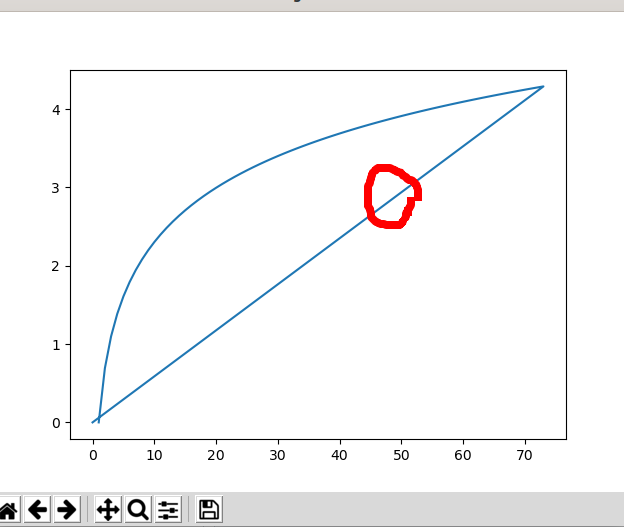I just don't need the straight line in the middle,but I don't kown how to correct it,and the following picture is my code: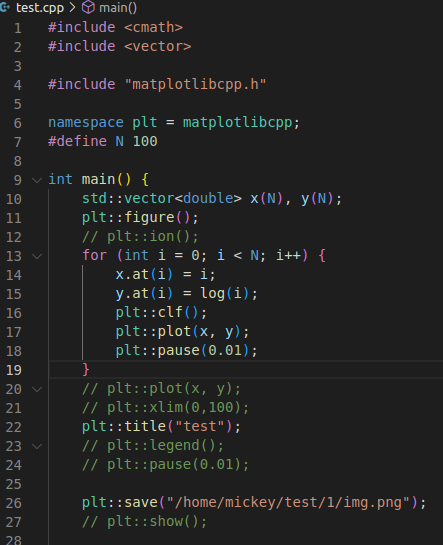And it's my first time to use matplotlibcpp, so I ask some help to handle the problem.

opened by Hit-Mickey 4
• #### Error LNK2019

I have the problem that after I linked all the include folders and libs folders the shown error appears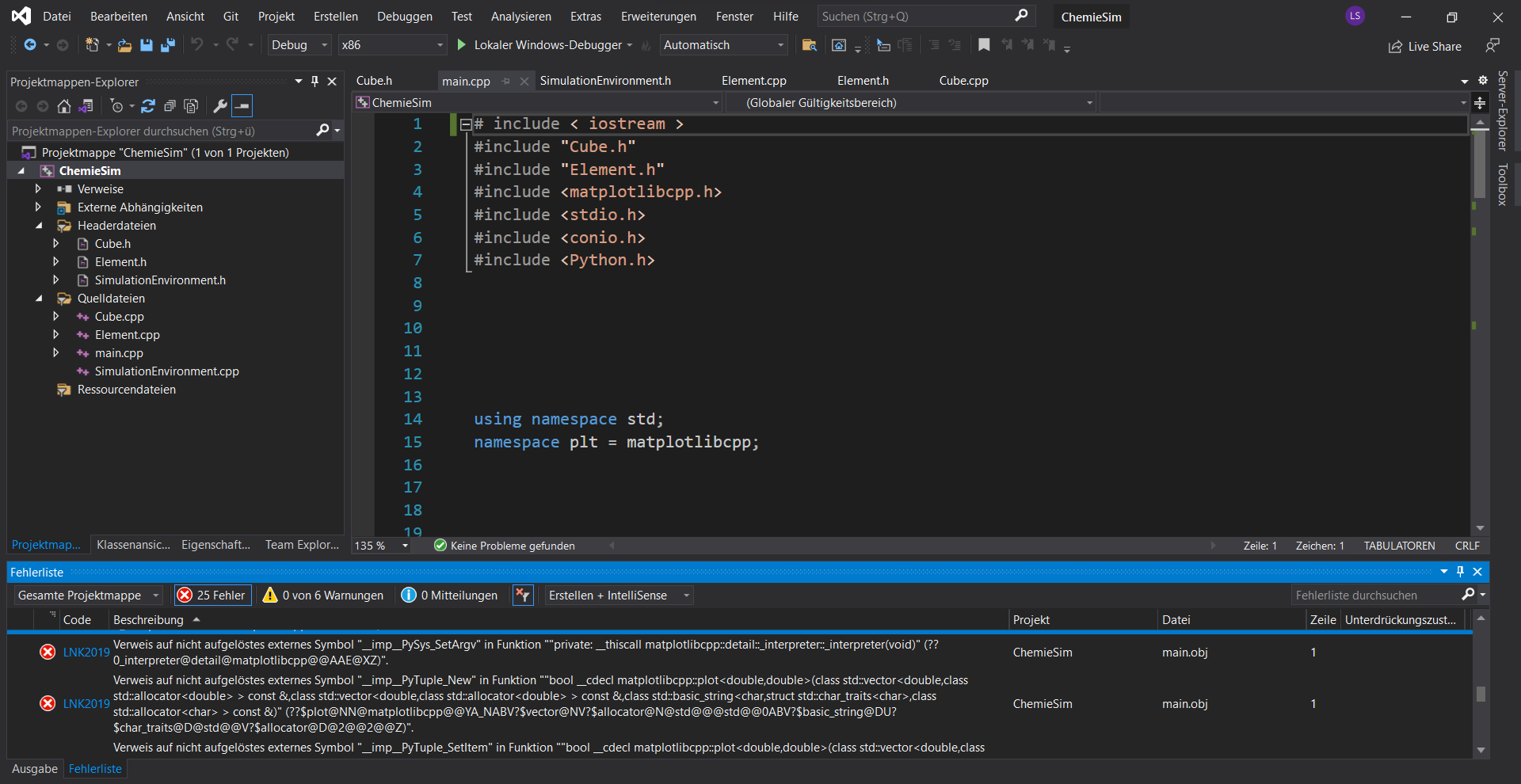I am coding in Visual studio 2019. The problem is I dont know where to even Search the mistake or what is missing. Thank you for your help

opened by lenovskyy 0
• #### Fix Debug runtime error Under the win32 platform.

Under the win32 platform and debug, python cannot find the matplotlib package normally. It is recommended that the debug definition include python.h is not applicable in this case.

opened by Polaris-cn 0
###### Benno Evers###### Header only, single file, simple and efficient C++ library to compute the signed distance function to a triangle mesh

TriangleMeshDistance Header only, single file, simple and efficient C++11 library to compute the signed distance function to a triangle mesh. The dist

100 Dec 28, 2022
###### A C++ header-only library of statistical distribution functions.

StatsLib StatsLib is a templated C++ library of statistical distribution functions, featuring unique compile-time computing capabilities and seamless

423 Jan 3, 2023
###### RcppFastFloat: Rcpp Bindings for the fastfloat C++ Header-Only Library

Converting ascii text into (floating-point) numeric values is a very common problem. The fast_float header-only C++ library by Daniel Lemire does this very well, and very fast at up to or over to 1 gigabyte per second as described in more detail in a recent arXiv paper.

18 Nov 15, 2022

dj_fft: Header-only FFT library Details This repository provides a header-only library to compute fourier transforms in 1D, 2D, and 3D. Its goal is to

134 Dec 29, 2022
###### C++ header-only fixed-point math library

fpm A C++ header-only fixed-point math library. "fpm" stands for "fixed-point math". It is designed to serve as a drop-in replacement for floating-poi

392 Jan 7, 2023
###### C++ header-only library with methods to efficiently encode/decode Morton codes in/from 2D/3D coordinates

Libmorton v0.2.7 Libmorton is a C++ header-only library with methods to efficiently encode/decode 64, 32 and 16-bit Morton codes and coordinates, in 2

488 Dec 31, 2022
###### A modern, C++20-native, single-file header-only dense 2D matrix library.

A modern, C++20-native, single-file header-only dense 2D matrix library. Contents Example usage creating matrices basic operations row, col, size, sha

62 Dec 17, 2022
###### A header-only C++ library for large scale eigenvalue problems

NOTE: Spectra 1.0.0 is released, with a lot of API-breaking changes. Please see the migration guide for a smooth transition to the new version. NOTE:

609 Jan 2, 2023
###### libmpc++ is a C++ header-only library to solve linear and non-linear MPC

libmpc++ libmpc++ is a C++ library to solve linear and non-linear MPC. The library is written in modern C++17 and it is tested to work on Linux, macOS

46 Dec 20, 2022
###### Header-only C++11 library to handle physical measures

cpp-measures Header-only C++11 library to handle physical measures License: This project is released under the Mozilla Public License 2.0. Purpose Thi

20 Jun 28, 2018
###### A work-in-progress C++20/23 header-only maths library for game development, embedded, kernel and general-purpose that works in constant context.

kMath /kmæθ/ A work-in-progress general-purpose C++20/23 header-only maths library that works in constant context Abstract The kMath Project aims to p

13 Sep 5, 2022
###### A C++ header only library for decomposition of spectra into a sum of response functions whose weights are positive definite.

DecompLib A C++ header only library for decomposition of spectra into a sum of response functions whose weights are positive definite. Introduction Bu

2 Jan 22, 2022
###### A matrix header-only library, uses graphs internally, helpful when your matrix is part of a simulation where it needs to grow many times (or auto expand)

GraphMat Header-only Library Matrix implemented as a graph, specially for the use case when it should be auto expanding at custom rate, specially in s

3 Oct 25, 2021
###### linalg.h is a single header, public domain, short vector math library for C++

linalg.h linalg.h is a single header, public domain, short vector math library for C++. It is inspired by the syntax of popular shading and compute la

758 Jan 7, 2023
###### nml is a simple matrix and linear algebra library written in standard C.

nml is a simple matrix and linear algebra library written in standard C.

45 Dec 9, 2022
###### Simple long integer math library for C++

SLIMCPP Simple long integer math library for C++ SLIMCPP is C++ header-only library that implements long integers that exceed maximum size of native t

23 Dec 1, 2022
###### A simple C++ complex & real matrix library, with matrix inversion, left division and determinant calculation

NaiveMatrixLib 帆帆的简易矩阵计算库 A simple C++ stdlib-based complex & real matrix library, with matrix inversion, left division (A\b) and determinant calculat

50 Dec 28, 2022
527 Jan 7, 2023
###### A C library for statistical and scientific computing

Apophenia is an open statistical library for working with data sets and statistical or simulation models. It provides functions on the same level as t

186 Sep 11, 2022